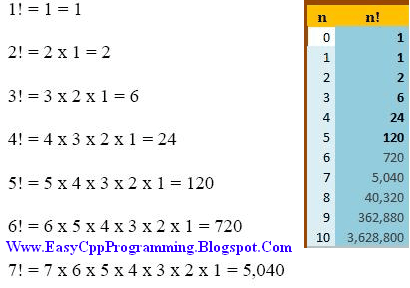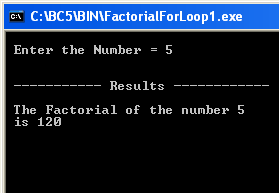# C++ Tutorial With Basic Programs for Beginners

C++ tutorial, C++ programs, C++ code, C++ flowcharts, C++ statements, C++ syntax, C++ examples

## Sunday, December 15, 2013

### Program Calculate Factorial of a Number

Today, we will learn the real logic behind C++ Program Factorial of a Given Number, in details.
In mathematics, the factorial of a non-negative integer n, denoted by n!, is the product of all positive integers less than or equal to n. For example,
5! = 5 x 4 x 3 x 2 x 1 = 120
Note: The value of 0! is 1.C++ Program Factorial of given number input by user at run time

### Source Code of Factorial of a Number program for DevC++ and other Modern Compilers / IDEs

```#include<iostream>

using namespace std;

int main()
{

int number, counter;
long int factorial;

cout<<"\n Enter the Number = ";
cin>>number;

factorial = 1;
for (counter = 1; counter <= number; counter++)
factorial = factorial * counter;

cout<<"\n\n ----------- Results ------------";

cout<<"\n\n The Factorial of the number "<<number<<"\n is "<<factorial;

return 0;

}
```

### A sample output:

Enter the Number = 5

----------- Results ------------

The Factorial of the number 5
is 120
--------------------------------
Process exited after 23.7 seconds with return value 0
Press any key to continue . . .

### Source Code for Turbo C++ 3.0 IDE

/*
Write a C++ Program to input a positive number
Calculate and Display Factorial of this number
by For Loop.

For Free C++ Programming Techniques (Example C++ Programs with Explainations), visit
Www.EasyCppProgramming.Blogspot.Com

and For Good Notes
Visit www.computergap.com
Visit   Www.ForFreeEducation.Blogspot.Com
*/
#include<iostream.h>

#include<conio.h>

void main()
{

clrscr();

int number, counter;
long int factorial;

cout<<"\n Enter the Number = ";

cin>>number;

factorial = 1;
for (counter = 1; counter <= number; counter++)
factorial = factorial * counter;

cout<<"\n\n ----------- Results ------------";

cout<<"\n\n The Factorial of the number "<<number<<"\n is "<<factorial;

getch();

}C++ Program to find Factorial of given Number

### Explain Logic of The Calculate Factorial of a Number, C++ Program

In the following lines, the above mentioned factorial program is further explained with the help of comments / use of documentation in the factorial program in C++

#### How Factorial of a Number in C++ Program Works:

#include<iostream.h>  //include header files, iostream is for cin, cout

#include<conio.h>   //include header files, conio.h is for clrscr();

void main()
{

clrscr();   //clear the output screen

int number, counter;   // declare variable number for input,
//counter is loop control counter variable
long int factorial;       // factorial may be a big number so declared as long

cout<<"\n Enter the Number = ";    // print message

cin>>number;       // input number

factorial = 1;         // intialize factorial to one

// now since 3!=1 x 2 x 3
// and n! = 1 x 2 x3 ..... x n, therfore factorial calculaton loop may be from 1 to n
// so that we can multiply all numbers from 1 to n
// and place the result of these products in factorial
for (counter = 1; counter <= number; counter++)
factorial = factorial * counter;

cout<<"\n\n ----------- Results ------------";  //print message

// print result of factorial program
cout<<"\n\n The Factorial of the number "<<number<<"\n is "<<factorial;

getch(); // to stay outpput screen as long as we press a key

}    // end

You can download and install Turbo C++ 3.0 Compiler here
You can read the use of Writing, compiling, executing programs in C++ in Turbo C++ here

# Download and Install Turbo C++ Full Screen Windows 7 Vista

You can find calculate factorial of a number program with while loop here
You can find calculate factorial of a number program with do while loop here
You can find calculate factorial of a number program using Recursion ( Recursive Factorial Function )

Share:

#### Post a Comment

We Love To Hear From You!